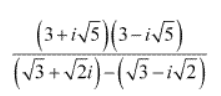NCERT Solutions for Class 11 Maths Chapter 5 Ex 5.1

# NCERT Solutions for Class 11 Maths Chapter 5 Ex 5.1

## NCERT Solutions for Class 11 Maths Chapter 5 Complex Numbers and Quadratic Equations Ex 5.1

NCERT Solutions for Class 11 Maths Chapter 5 Complex Numbers and Quadratic Equations Ex 5.1 are the part of NCERT Solutions for Class 11 Maths. Here you can find the NCERT Solutions for Class 11 Maths chapter 5 Complex Numbers and Quadratic Equations Ex 5.1.

Express each of the complex number given in the Exercises 1 to 10 in the form a + ib.

#### Ex 5.1 Class 11 Maths Question 1.

(5i)(−3i/5)

Solution.
(5i)(−i3/5)
= -3i2 = -3(-1)                    [
i2 = -1]
= 3 = 3 + 0i

#### Ex 5.1 Class 11 Maths Question 2.

i9 + i19

Solution.

i9 + i19 = (i2)4.i + (i2)9.i = (-1)4.i + (-1)9.i

= i + (-1)i = i – i = 0 = 0 + 0i

i-39

Solution.

#### Ex 5.1 Class 11 Maths Question 4.

3(7 + i7) + i(7 + i7)

Solution.
3(7 + i7) + i(7 + i7) = 21 + 21i + 7i + 7i2
= 21 + (21 + 7)i + (-1)7 = 21 – 7 + 28i
= 14 + 28i

#### Ex 5.1 Class 11 Maths Question 5.

(1 – i) – (-1 + i6)

Solution.
(1 – i) – (-1 + i6) = 1 – i + 1 – 6i
= (1 + 1) – i(1 + 6)
= 2 – 7i

Solution.

Solution.

#### Ex 5.1 Class 11 Maths Question 8.

(1 – i)4

Solution.
(1 – i)4 = [(1 – i)2]2 = [1 – 2i + i2]2
= [1 – 2i + (-1)]2
= (-2i)2 = 4i2 = 4(-1) = –4
= –4 + 0i

(1/3 + 3i)3

Solution.

#### Ex 5.1 Class 11 Maths Question 10.

(−2 1/3 i)3

Solution.

Find the multiplicative inverse of each of the complex numbers given in the Exercises 11 to 13.

4 – 3i

Solution.

5 + 3i

Solution.

-i

Solution.

#### Ex 5.1 Class 11 Maths Question 14.

Express the following expression in the form of a + ib:Solution.
We have,